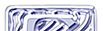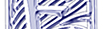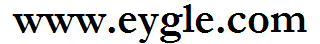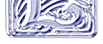首页 技术基础 备份恢复 SQL优化 诊断案例 BLOG 留言板 服务 生活 网摘 阅读 下载 智能巡检 English

### 对于SYS.ALL_SYNONYMS查询的性能问题Oracle 10g之后，对于ALL_SYNONYMS的查询可能带来问题，以下是摘录。

In this Document

## Applies to:

Oracle Server - Enterprise Edition - Version: 10.2.0.1 to 10.2.0.4 - Release: 10.2 to 10.2
Information in this document applies to any platform.

## Symptoms

In some cases queries against the ALL_SYNONYMS view are slower after upgrading to 10.2.0.x
For example:

```SELECT /*+ RULE */ COUNT(*) FROM ALL_SYNONYMS WHERE OWNER='PUBLIC' AND SYNONYM_NAME='&1'```

Oracle 10g Release 10.2:

```
call     count       cpu    elapsed       disk      query    current        rows
------- ------  -------- ---------- ---------- ---------- ----------  ----------
Parse       81      0.02       0.00          0          0          0           0
Execute     81      0.05       0.09          0          0          0           0
Fetch       81    355.93     371.05          0   45269282          0          81
------- ------  -------- ---------- ---------- ---------- ----------  ----------
total      243    356.00     371.15          0   45269282          0          81
Oracle 9i Release 9.2:```
``````call     count       cpu    elapsed       disk      query    current        rows
------- ------  -------- ---------- ---------- ---------- ----------  ----------
Parse       81      0.02       0.02          0          0          0           0
Execute     81      0.04       0.01          0          0          0           0
Fetch       81      0.03       0.01          0        810          0          81
------- ------  -------- ---------- ---------- ---------- ----------  ----------
total      243      0.09       0.05          0        810          0          81```
```

## Changes

Changed to a version higher than 9.2 from 9.2 or below.

## Cause

10g release 10.1.0.5, 10.2.0.1 and above contain a new view definition for ALL_SYNONYMS which is the result of a fix to an outstanding defect. This defect meant that certain synonyms would not be displayed when selecting from ALL_SYNONYMS. To fix the defect a new, more complex, ALL_SYNONYMS was required and as a side affect queries that select against the new ALL_SYNONYMS definition have much more complicated execution plans and may be more expensive when compared to execution plans in earlier versions (such as 9.2).
For example:

Compare the execution plan for ALL_SYNONYMS queries in 10046 trace file in 10.2 and in 9.2, to confirm execution plan is more resource consuming in 10.2 :

In 9.2 execution plan is :

```Rows     Row Source Operation
-------  ---------------------------------------------------
1  SORT AGGREGATE
1   FILTER
1    NESTED LOOPS
1     NESTED LOOPS
1      TABLE ACCESS BY INDEX ROWID USER\$
1       INDEX UNIQUE SCAN I_USER1 (object id 41)
1      TABLE ACCESS BY INDEX ROWID OBJ\$
1       INDEX RANGE SCAN I_OBJ2 (object id 34)
1     TABLE ACCESS BY INDEX ROWID SYN\$
1      INDEX UNIQUE SCAN I_SYN1 (object id 98)
0    FILTER
0     NESTED LOOPS
0      NESTED LOOPS
0       TABLE ACCESS BY INDEX ROWID USER\$
0        INDEX UNIQUE SCAN I_USER1 (object id 41)
0       TABLE ACCESS BY INDEX ROWID OBJ\$
0        INDEX RANGE SCAN I_OBJ2 (object id 34)
0      INDEX RANGE SCAN I_OBJAUTH1 (object id 100)
0     FIXED TABLE FULL X\$KZSRO
0    FIXED TABLE FULL X\$KZSPR```

In 10.2 execution plan is :

```Rows     Row Source Operation
-------  ---------------------------------------------------
1  SORT AGGREGATE (cr=558882 pr=0 pw=0 time=4326033 us)
1   VIEW  ALL_SYNONYMS (cr=558882 pr=0 pw=0 time=4325993 us)
1    SORT UNIQUE (cr=558882 pr=0 pw=0 time=4325988 us)
1     UNION-ALL  (cr=558882 pr=0 pw=0 time=4325909 us)
1      FILTER  (cr=10 pr=0 pw=0 time=185 us)
1       NESTED LOOPS  (cr=10 pr=0 pw=0 time=173 us)
1        NESTED LOOPS  (cr=7 pr=0 pw=0 time=136 us)
1         TABLE ACCESS BY INDEX ROWID USER\$ (cr=3 pr=0 pw=0 time=56 us)
1          INDEX UNIQUE SCAN I_USER1 (cr=2 pr=0 pw=0 time=39 us)(object id 41)
1         TABLE ACCESS BY INDEX ROWID OBJ\$ (cr=4 pr=0 pw=0 time=77 us)
1          INDEX RANGE SCAN I_OBJ2 (cr=3 pr=0 pw=0 time=48 us)(object id 34)
1        TABLE ACCESS BY INDEX ROWID SYN\$ (cr=3 pr=0 pw=0 time=31 us)
1         INDEX UNIQUE SCAN I_SYN1 (cr=2 pr=0 pw=0 time=17 us)(object id 98)
0       FILTER  (cr=0 pr=0 pw=0 time=0 us)
0        FILTER  (cr=0 pr=0 pw=0 time=0 us)
0         NESTED LOOPS  (cr=0 pr=0 pw=0 time=0 us)
0          NESTED LOOPS  (cr=0 pr=0 pw=0 time=0 us)
0           TABLE ACCESS BY INDEX ROWID USER\$ (cr=0 pr=0 pw=0 time=0 us)
0            INDEX UNIQUE SCAN I_USER1 (cr=0 pr=0 pw=0 time=0 us)(object id 41)
0           TABLE ACCESS BY INDEX ROWID OBJ\$ (cr=0 pr=0 pw=0 time=0 us)
0            INDEX RANGE SCAN I_OBJ2 (cr=0 pr=0 pw=0 time=0 us)(object id 34)
0          INDEX RANGE SCAN I_OBJAUTH1 (cr=0 pr=0 pw=0 time=0 us)(object id 100)
0        FIXED TABLE FULL X\$KZSRO (cr=0 pr=0 pw=0 time=0 us)
0       FIXED TABLE FULL X\$KZSPR (cr=0 pr=0 pw=0 time=0 us)
0      NESTED LOOPS  (cr=558872 pr=0 pw=0 time=4325697 us)
0       NESTED LOOPS  (cr=558872 pr=0 pw=0 time=4325692 us)
7        NESTED LOOPS  (cr=558849 pr=0 pw=0 time=4325568 us)
1         TABLE ACCESS BY INDEX ROWID USER\$ (cr=3 pr=0 pw=0 time=25 us)
1          INDEX UNIQUE SCAN I_USER1 (cr=2 pr=0 pw=0 time=15 us)(object id 41)
7         VIEW  _ALL_SYNONYMS_TREE (cr=558846 pr=0 pw=0 time=4325543 us)
7          CONNECT BY WITHOUT FILTERING (cr=558846 pr=0 pw=0 time=4325527 us)
7           FILTER  (cr=279521 pr=0 pw=0 time=1848969 us)
18            COUNT  (cr=279323 pr=0 pw=0 time=1505655 us)
18             NESTED LOOPS  (cr=279323 pr=0 pw=0 time=1143804 us)
25717              NESTED LOOPS  (cr=201352 pr=0 pw=0 time=1113824 us)
27395               NESTED LOOPS  (cr=148330 pr=0 pw=0 time=925979 us)
69679                NESTED LOOPS  (cr=51254 pr=0 pw=0 time=487918 us)
958                 TABLE ACCESS FULL USER\$ (cr=233 pr=0 pw=0 time=2996 us)
69679                 TABLE ACCESS BY INDEX ROWID OBJ\$ (cr=51021 pr=0 pw=0 time=431685 us)
69679                  INDEX RANGE SCAN I_OBJ2 (cr=3216 pr=0 pw=0 time=80937 us)(object id 34)
27395                TABLE ACCESS BY INDEX ROWID SYN\$ (cr=97076 pr=0 pw=0 time=697424 us)
27395                 INDEX UNIQUE SCAN I_SYN1 (cr=69681 pr=0 pw=0 time=425927 us)(object id 98)
25717               TABLE ACCESS BY INDEX ROWID USER\$ (cr=53022 pr=0 pw=0 time=309207 us)
25717                INDEX UNIQUE SCAN I_USER1 (cr=27305 pr=0 pw=0 time=150664 us)(object id 41)
18              TABLE ACCESS BY INDEX ROWID OBJ\$ (cr=77971 pr=0 pw=0 time=598777 us)
26615               INDEX RANGE SCAN I_OBJ2 (cr=51865 pr=0 pw=0 time=371282 us)(object id 34)
7            FILTER  (cr=198 pr=0 pw=0 time=1794 us)
7             NESTED LOOPS  (cr=198 pr=0 pw=0 time=1489 us)
50              NESTED LOOPS  (cr=91 pr=0 pw=0 time=853 us)
7               NESTED LOOPS  (cr=52 pr=0 pw=0 time=414 us)
7                TABLE ACCESS BY INDEX ROWID SYN\$ (cr=31 pr=0 pw=0 time=264 us)
7                 INDEX UNIQUE SCAN I_SYN1 (cr=24 pr=0 pw=0 time=179 us)(object id 98)
7                TABLE ACCESS BY INDEX ROWID USER\$ (cr=21 pr=0 pw=0 time=126 us)
7                 INDEX UNIQUE SCAN I_USER1 (cr=14 pr=0 pw=0 time=74 us)(object id 41)
50               TABLE ACCESS BY INDEX ROWID OBJ\$ (cr=39 pr=0 pw=0 time=320 us)
50                INDEX RANGE SCAN I_OBJ2 (cr=22 pr=0 pw=0 time=215 us)(object id 34)
7              INDEX RANGE SCAN I_OBJAUTH1 (cr=107 pr=0 pw=0 time=596 us)(object id 100)
2             FIXED TABLE FULL X\$KZSRO (cr=0 pr=0 pw=0 time=174 us)
18           COUNT  (cr=279325 pr=0 pw=0 time=1440703 us)
18            NESTED LOOPS  (cr=279325 pr=0 pw=0 time=1440701 us)
25717             NESTED LOOPS  (cr=201354 pr=0 pw=0 time=1112712 us)
27395              NESTED LOOPS  (cr=148332 pr=0 pw=0 time=926537 us)
69679               NESTED LOOPS  (cr=51255 pr=0 pw=0 time=487858 us)
958                TABLE ACCESS FULL USER\$ (cr=233 pr=0 pw=0 time=2945 us)
69679                TABLE ACCESS BY INDEX ROWID OBJ\$ (cr=51022 pr=0 pw=0 time=431397 us)
69679                 INDEX RANGE SCAN I_OBJ2 (cr=3216 pr=0 pw=0 time=80879 us)(object id 34)
27395               TABLE ACCESS BY INDEX ROWID SYN\$ (cr=97077 pr=0 pw=0 time=687683 us)
27395                INDEX UNIQUE SCAN I_SYN1 (cr=69681 pr=0 pw=0 time=415512 us)(object id 98)
25717              TABLE ACCESS BY INDEX ROWID USER\$ (cr=53022 pr=0 pw=0 time=308614 us)
25717               INDEX UNIQUE SCAN I_USER1 (cr=27305 pr=0 pw=0 time=150815 us)(object id 41)
18             TABLE ACCESS BY INDEX ROWID OBJ\$ (cr=77971 pr=0 pw=0 time=591777 us)
26615              INDEX RANGE SCAN I_OBJ2 (cr=51865 pr=0 pw=0 time=363738 us)(object id 34)
7           FILTER  (cr=198 pr=0 pw=0 time=1794 us)
7             NESTED LOOPS  (cr=198 pr=0 pw=0 time=1489 us)
50              NESTED LOOPS  (cr=91 pr=0 pw=0 time=853 us)
7               NESTED LOOPS  (cr=52 pr=0 pw=0 time=414 us)
7                TABLE ACCESS BY INDEX ROWID SYN\$ (cr=31 pr=0 pw=0 time=264 us)
7                 INDEX UNIQUE SCAN I_SYN1 (cr=24 pr=0 pw=0 time=179 us)(object id 98)
7                TABLE ACCESS BY INDEX ROWID USER\$ (cr=21 pr=0 pw=0 time=126 us)
7                 INDEX UNIQUE SCAN I_USER1 (cr=14 pr=0 pw=0 time=74 us)(object id 41)
50               TABLE ACCESS BY INDEX ROWID OBJ\$ (cr=39 pr=0 pw=0 time=320 us)
50                INDEX RANGE SCAN I_OBJ2 (cr=22 pr=0 pw=0 time=215 us)(object id 34)
7              INDEX RANGE SCAN I_OBJAUTH1 (cr=107 pr=0 pw=0 time=596 us)(object id 100)
2            FIXED TABLE FULL X\$KZSRO (cr=0 pr=0 pw=0 time=174 us)
0        TABLE ACCESS BY INDEX ROWID OBJ\$ (cr=0 pr=0 pw=0 time=0 us)
0         INDEX UNIQUE SCAN I_OBJ1 (cr=0 pr=0 pw=0 time=0 us)(object id 33)
0       TABLE ACCESS BY INDEX ROWID SYN\$ (cr=0 pr=0 pw=0 time=0 us)
0        INDEX UNIQUE SCAN I_SYN1 (cr=0 pr=0 pw=0 time=0 us)(object id 98)```

## Solution

There is no resolution to this issue.
In future versions it is possible that this may be addressed but any fix would not be back-portable to previous versions as architechtural changes are required to enable it.

The current ALL_SYNONYMS view's changes and additional explain plan steps are necessary to resolve the original issue with the ALL_SYNONYMS view.

To have similar performance from the ALL_SYNONYMS view on 10g to version 9.2 do the following steps :

1. Create a new view "SYS.ALL_SYNONYMS_920X" with all_synonyms definition from database 920X. The view definition for ALL_SYNONYMS is stored in the \$ORACLE_HOME/rdbms/admin/catalog.sql script. To create the new view, login as the SYS user and execute the following:
```connect / as sysdba create or replace view SYS.ALL_SYNONYMS_920X (OWNER, SYNONYM_NAME, TABLE_OWNER, TABLE_NAME, DB_LINK) as select u.name, o.name, s.owner, s.name, s.node from sys.user\$ u, sys.syn\$ s, sys.obj\$ o where o.obj# = s.obj# and o.type# = 5 and o.owner# = u.user# and ( o.owner# in (USERENV('SCHEMAID'), 1 /* PUBLIC */) /* user's private, any public */ or /* user has any privs on base object */ exists (select null from sys.objauth\$ ba, sys.obj\$ bo, sys.user\$ bu where bu.name = s.owner and bo.name = s.name and bu.user# = bo.owner# and ba.obj# = bo.obj# and ( ba.grantee# in (select kzsrorol from x\$kzsro) or ba.grantor# = USERENV('SCHEMAID') ) ) or /* user has system privileges */ exists (select null from v\$enabledprivs where priv_number in (-45 /* LOCK ANY TABLE */, -47 /* SELECT ANY TABLE */, -48 /* INSERT ANY TABLE */, -49 /* UPDATE ANY TABLE */, -50 /* DELETE ANY TABLE */) ) ) /```
2. To force users to select from this new view without code modification, a private synonym can be created:
````CREATE SYNONYM username.ALL_SYNONYMS FOR SYS.ALL_SYNONYMS_920X `
```

This is private synonym must be created for any user executing which wants to use the new definition.
Note that the users could also select from the new definition directly but this would require code references to be changed in the application.

Note that this workaround simply reverts to the previous view definition.It simply provides a workaround to achieve the old performance in the short term.

Having reverted to the previous view definition users may hit Bug:3369744 per which ALL_SYNONYMS does not show all accessible synonyms.

## References

BUG:5454590 - SELECT FROM ALL_SYNONYMS AND ALL_OBJECTS IS MUCH SLOWER IN 10.2.0.2
NOTE:364822.1 - Poor Performance On Certain Dictionary Queries After Upgrade To 10g
SR:16161618.6

>> 2007-11-16文章:

By eygle on 2011-11-16 17:54 | Comments (0) | Oracle摘 | 2899 |